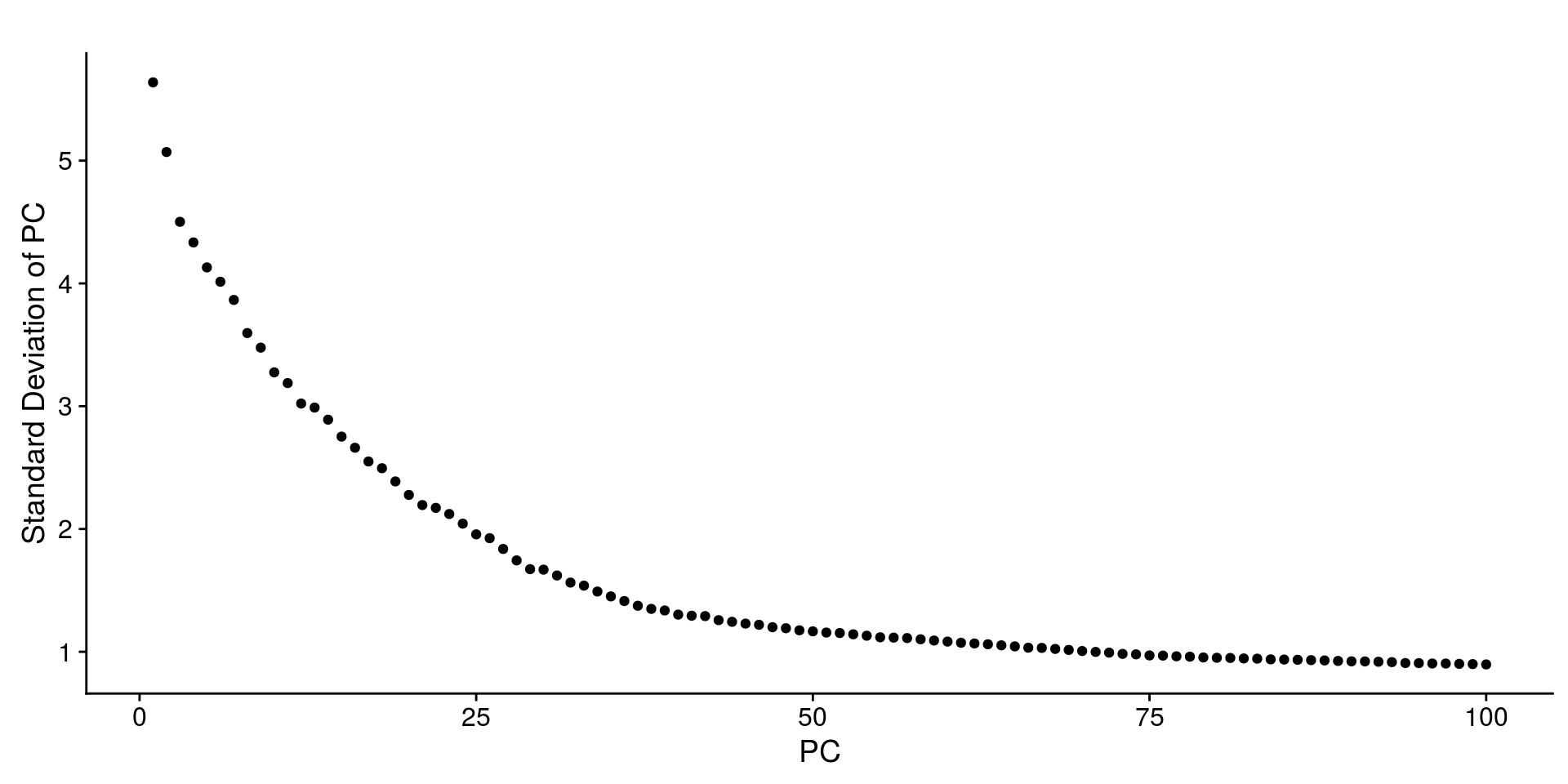In the following tutorial, we examine the recently published Microwell-seq “Mouse Cell Atlas”, composed of hundreds of thousands of cells derived from all major mouse organs. For those that are getting started using Seurat, we recommend first working through our 3k PBMC tutorial, which introduces the basic functionality of the package.

Our goal is to demonstrate a workflow for handling very large datasets in Seurat, emphasizing recent improvements we have made for speed and memory efficiency. We do not perform downstream biological analyses on the resulting clusters, but encourage users to explore this dataset and interpret this exciting resource. All analyses here are performed in memory, but we also now support storage on-disk (using the HDF5-based loom framework). See this vignette for a workflow of the same MCA dataset using loomR.

### Setup the Seurat Object

The original data for the MCA is available here. For ease of getting started, we provide a sparse matrix R data file (.rds) file containing the combined expression matrix and the published metadata file here.

``````library(Matrix)
library(Seurat)
row.names = 1)``````

We will analyze ~242,000 cells that were assigned a cluster ID in the original study. As a result, we don’t do perform additional QC steps or filtration steps here.

``````mca <- CreateSeuratObject(raw.data = mca.matrix, meta.data = mca.metadata, project = "MouseCellAtlas")
# Only keep annotated cells
mca <- SubsetData(mca, cells.use = rownames(mca@meta.data[!is.na(mca@meta.data\$ClusterID),
]), do.clean = TRUE)
# Leaves us with 242k cells
mca``````
``````## An object of class seurat in project MouseCellAtlas
##  39855 genes across 242533 samples.``````

### Data Preprocessing

We perform standard log-normalization.

``mca <- NormalizeData(object = mca, normalization.method = "LogNormalize", scale.factor = 10000)``

`FindVariableGenes` calculates the variance and mean for each gene in the dataset in the dataset (storing this in `object@hvg.info`), and sorts genes by their variance/mean ratio (VMR). We have observed that for large-cell datasets with unique molecular identifiers, selecting highly variable genes (HVG) simply based on VMR is an efficient and robust strategy. Here, we select the top 1,000 HVG for downstream analysis.

``````mca <- FindVariableGenes(object = mca, mean.function = ExpMean, dispersion.function = LogVMR,
do.plot = FALSE)

We calculate and regress out mitochondrial expression per cell.

Suggestions for large datasets
• ScaleData now has the option for multi-core parallel processing, using the `num.cores` argument. Thanks to aymanm for this improvement.
• You can perform gene scaling on only the HVG, dramatically improving speed and memory use. Since dimensional reduction is run only on HVG, this will not affect downstream results.
``````mito.genes <- grep(pattern = "^mt-", x = rownames(x = mca@data), value = TRUE)
percent.mito <- Matrix::colSums(mca@raw.data[mito.genes, ])/Matrix::colSums(mca@raw.data)
mca <- ScaleData(object = mca, genes.use = hv.genes, display.progress = FALSE,
vars.to.regress = "percent.mito", do.par = TRUE, num.cores = 4)``````

### Dimensional Reduction (PCA)

``````mca <- RunPCA(object = mca, pc.genes = hv.genes, pcs.compute = 100, do.print = TRUE,
pcs.print = 1:5, genes.print = 5)``````
``````##  "PC1"
##  "Prm1"  "Prm2"  "Meig1" "Fabp9" "Ldhc"
##  ""
##  "Tmsb4x" "B2m"    "Fth1"   "Ftl1"   "Rpl18a"
##  ""
##  ""
##  "PC2"
##  "Sparc"  "Col2a1" "Col9a2" "Col9a1" "Col9a3"
##  ""
##  "Defa24" "Reg3b"  "Defa30" "Igkc"   "Reg3g"
##  ""
##  ""
##  "PC3"
##  "Camp"   "Ngp"    "S100a9" "S100a8" "Chil3"
##  ""
##  "Defa24" "Reg3b"  "Defa30" "Reg3g"  "Defa17"
##  ""
##  ""
##  "PC4"
##  "Csn1s2b" "Wap"     "Trf"     "Lalba"   "Glycam1"
##  ""
##  "S100a8" "S100a9" "Lyz2"   "Tyrobp" "Srgn"
##  ""
##  ""
##  "PC5"
##  "Wap"     "Csn1s2b" "Lalba"   "Glycam1" "Csn1s2a"
##  ""
##  "Tmsb10" "Rplp1"  "Rpl13"  "Rps27"  "Rps15a"
##  ""
##  ""``````
Suggestions for large datasets
• To select downstream PCs for analysis, `JackStraw` now also features mutli-core parallelization. However, for data sets of this size, the saturation of explained variance, along with the visualization of PC ‘metagenes’, are likely to be more effective. We select 75 PCs here for downstream analysis.
``PCElbowPlot(object = mca, num.pc = 100)````PCHeatmap(mca, pc.use = c(1:3, 70:75), cells.use = 500, do.balanced = TRUE)``